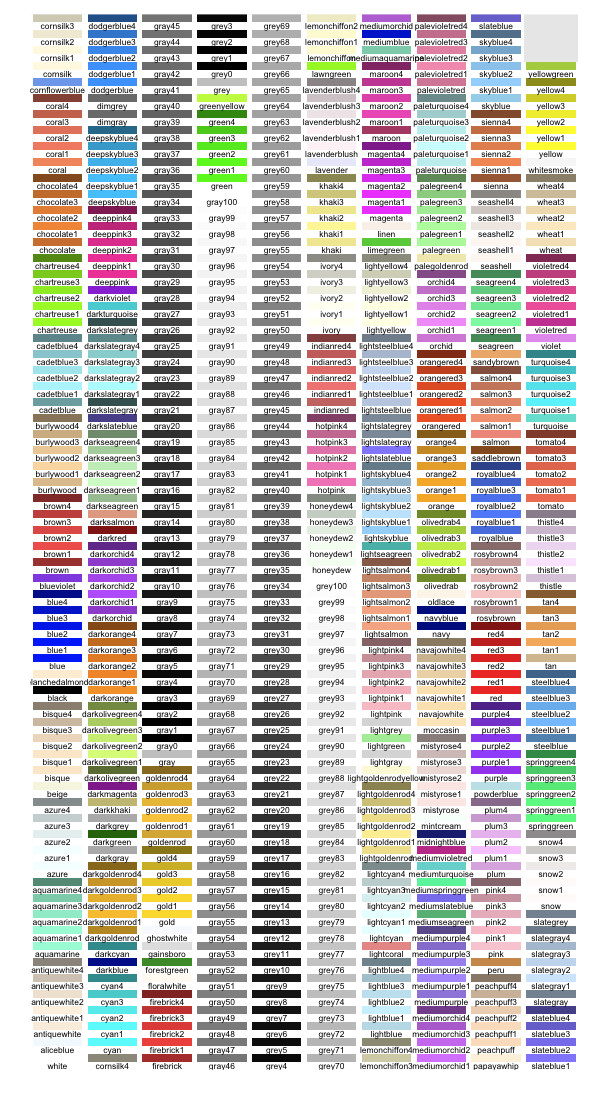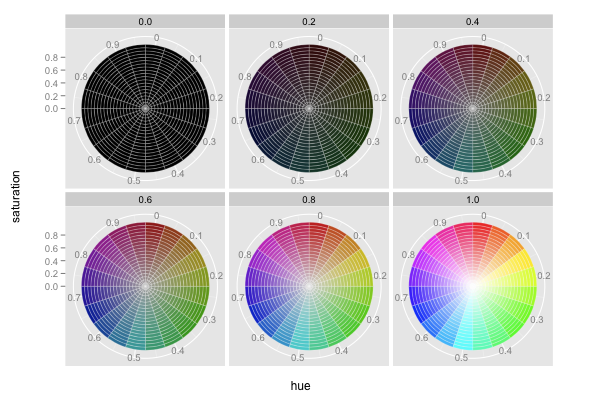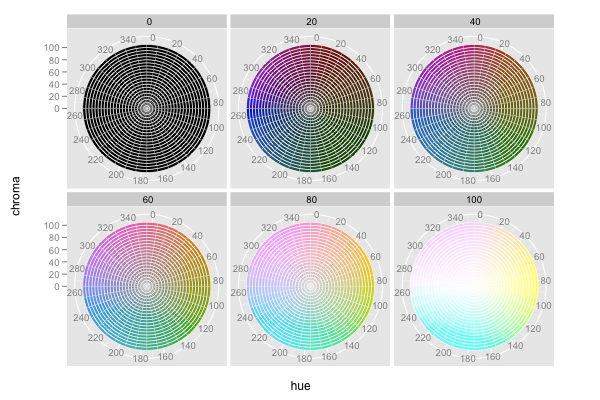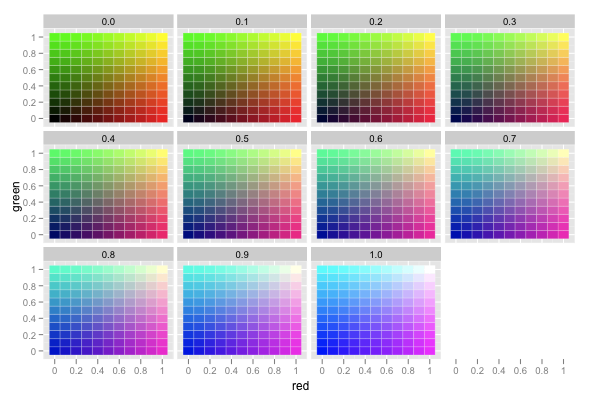## ggplot2 Quick Reference: colour (and fill)

### Specifying Colours

In R, a colour is represented as a string (see Color Specification section of the R `par()` function). Basically, a colour is defined, like in HTML/CSS, using the hexadecimal values (00 to FF) for red, green, and blue, concatenated into a string, prefixed with a "#". A pure red colour this is represented with "#FF0000".

#### Predefined Colour Names

Besides the "#RRGGBB" RGB colour strings, one can also use one of R's predefined named colours:```d=data.frame(c=colors(), y=seq(0, length(colors())-1)%%66, x=seq(0, length(colors())-1)%/%66)
ggplot() +
scale_x_continuous(name="", breaks=NA, expand=c(0, 0)) +
scale_y_continuous(name="", breaks=NA, expand=c(0, 0)) +
scale_fill_identity() +
geom_rect(data=d, mapping=aes(xmin=x, xmax=x+1, ymin=y, ymax=y+1), fill="white") +
geom_rect(data=d, mapping=aes(xmin=x+0.05, xmax=x+0.95, ymin=y+0.5, ymax=y+1, fill=c)) +
geom_text(data=d, mapping=aes(x=x+0.5, y=y+0.5, label=c), colour="black", hjust=0.5, vjust=1, size=3)```

#### Hue Saturation Value (HSV) = Hue Saturation Brightness (HSB) Colour Space

A colour can be specified using R's hsv() function that takes three arguments: hue, saturation, and value (brightness), all in the range [0, 1].```d=expand.grid(h=seq(0,0.95,0.05), s=seq(0,0.95,0.05), v=seq(0,1,0.2))
ggplot() +
coord_polar(theta="x") +
facet_wrap(~v) +
scale_x_continuous(name="hue", limits=c(0,1), breaks=seq(0.025,0.925,0.1), labels=seq(0,0.9,0.1)) +
scale_y_continuous(name="saturation", breaks=seq(0, 1, 0.2)) +
scale_fill_identity() +
geom_rect(data=d, mapping=aes(xmin=h, xmax=h+resolution(h), ymin=s, ymax=s+resolution(s), fill=hsv(h,s,v)), color="white", size=0.1)```

#### Hue Chroma Luminance (HCL) Colour Space

A colour can be specified using R's hcl() function that takes three arguments: hue [0,360], chroma [0,100], and luminance [0,100]. This space is similar to the HSV space, however, in the HCL space steps of equal size correspond to approximately equal perceptual changes in colour. Note that the possible values of chroma and luminance actually depend on the specific hue. The ranges above are only indicative.```d=expand.grid(h=seq(0,350,10), c=seq(0,100,5), l=seq(0,100,20))
ggplot() +
coord_polar(theta="x")+facet_wrap(~l) +
scale_x_continuous(name="hue", limits=c(0,360), breaks=seq(5,345,20), labels=seq(0,340,20)) +
scale_y_continuous(name="chroma", breaks=seq(0, 100, 20)) +
scale_fill_identity() +
geom_rect(data=d, mapping=aes(xmin=h, xmax=h+resolution(h), ymin=c, ymax=c+resolution(c), fill=hcl(h,c,l)), color="white", size=0.1)```

#### Red Green Blue (RGB) Colour Space

RGB is the built-in colour space. Instead of "manually" creating a #RRGGBB colour string, a colour can be specified using R's rgb() function that takes three arguments: red, green, and blue (which, by default, all have a range of [0, 1]).```d=expand.grid(r=seq(0,1,0.1), g=seq(0,1,0.1), b=seq(0,1,0.1))
ggplot() +
facet_wrap(~b) +
scale_x_continuous(name="red", breaks=seq(0.05, 1.05, 0.2), labels=seq(0, 1, 0.2)) +
scale_y_continuous(name="green", breaks=seq(0.05, 1.05, 0.2), labels=seq(0, 1, 0.2)) +
scale_fill_identity() +
geom_rect(data=d, mapping=aes(xmin=r, xmax=r+resolution(r), ymin=g, ymax=g+resolution(g), fill=rgb(r,g,b)), color="white", size=0.1)```

For best visual performance, we recommend mapping data onto the hue of a colour (using the HSV or HCL colour spaces).